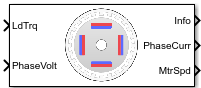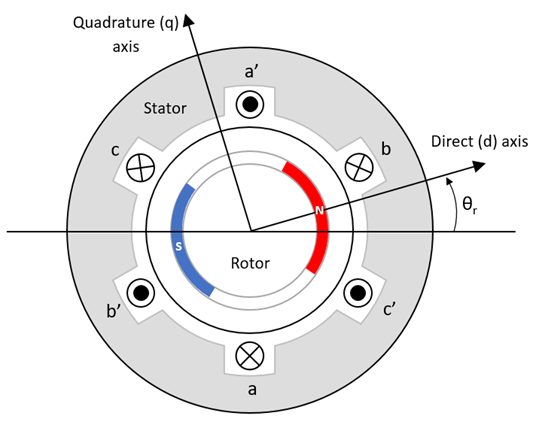Documentation

# Interior PMSM

Three-phase interior permanent magnet synchronous motor with sinusoidal back electromotive force

• Library:
• Powertrain Blockset / Propulsion / Electric Motors## Description

The Interior PMSM block implements a three-phase interior permanent magnet synchronous motor (PMSM) with sinusoidal back electromotive force. The block uses the three-phase input voltages to regulate the individual phase currents, allowing control of the motor torque or speed.

By default, the block sets the Simulation type parameter to `Continuous` to use a continuous sample time during simulation. If you want to generate code for fixed-step double- and single-precision targets, considering setting the parameter to `Discrete`. Then specify a Sample Time, Ts parameter.

On the Parameters tab, if you select `Back-emf`, the block implements this equation to calculate the permanent flux linkage constant.

`${\lambda }_{pm}=\frac{1}{\sqrt{3}}\cdot \frac{{K}_{e}}{1000P}\cdot \frac{60}{2\pi }$`

### Motor Construction

This figure shows the motor construction with a single pole pair on the motor.The motor magnetic field due to the permanent magnets creates a sinusoidal rate of change of flux with motor angle.

For the axes convention, the a-phase and permanent magnet fluxes are aligned when motor angle θr is zero.

### Three-Phase Sinusoidal Model Electrical System

The block implements these equations, expressed in the motor flux reference frame (dq frame). All quantities in the motor reference frame are referred to the stator.

`$\begin{array}{l}{\omega }_{e}=P{\omega }_{m}\\ \frac{d}{dt}{i}_{d}=\frac{1}{{L}_{d}}{v}_{d}-\frac{R}{{L}_{d}}{i}_{d}+\frac{{L}_{q}}{{L}_{d}}P{\omega }_{m}{i}_{q}\end{array}$`

`$\frac{d}{dt}{i}_{q}=\frac{1}{{L}_{q}}{v}_{q}-\frac{R}{{L}_{q}}{i}_{q}-\frac{{L}_{d}}{{L}_{q}}P{\omega }_{m}{i}_{d}-\frac{{\lambda }_{pm}P{\omega }_{m}}{{L}_{q}}$`

`${T}_{e}=1.5P\left[{\lambda }_{pm}{i}_{q}+\left({L}_{d}-{L}_{q}\right){i}_{d}{i}_{q}\right]$`

The Lq and Ld inductances represent the relation between the phase inductance and the motor position due to the saliency of the motor.

The equations use these variables.

 Lq, Ld q- and d-axis inductances R Resistance of the stator windings iq, id q- and d-axis currents vq, vd q- and d-axis voltages ωm Angular mechanical velocity of the motor ωe Angular electrical velocity of the motor λpm Permanent flux linkage constant Ke Back electromotive force (EMF) P Number of pole pairs Te Electromagnetic torque Θe Electrical angle

### Mechanical System

The motor angular velocity is given by:

`$\begin{array}{c}\frac{d}{dt}{\omega }_{m}=\frac{1}{J}\left({T}_{e}-{T}_{f}-F{\omega }_{m}-{T}_{m}\right)\\ \frac{d{\theta }_{m}}{dt}={\omega }_{m}\end{array}$`

The equations use these variables.

 J Combined inertia of motor and load F Combined viscous friction of motor and load θm Motor mechanical angular position Tm Motor shaft torque Te Electromagnetic torque Tf Motor shaft static friction torque ωm Angular mechanical velocity of the motor

### Power Accounting

For the power accounting, the block implements these equations.

Bus Signal DescriptionVariableEquations

`PwrInfo`

`PwrTrnsfrd` — Power transferred between blocks

• Positive signals indicate flow into block

• Negative signals indicate flow out of block

`PwrMtr`

Mechanical power

Pmot

`PwrBus`

Electrical power

Pbus

`PwrNotTrnsfrd` — Power crossing the block boundary, but not transferred

• Positive signals indicate an input

• Negative signals indicate a loss

`PwrElecLoss`

Resistive power loss

Pelec

`PwrMechLoss`

Mechanical power loss

Pmech

When Port Configuration is set to `Torque`:

When Port Configuration is set to `Speed`:

`PwrStored` — Stored energy rate of change

• Positive signals indicate an increase

• Negative signals indicate a decrease

`PwrMtrStored`

Stored motor power

Pstr

The equations use these variables.

 Rs Stator resistance ia, ib, ic Stator phase a, b, and c current isq, isd Stator q- and d-axis currents van, vbn, vcn Stator phase a, b, and c voltage ωm Angular mechanical velocity of the rotor F Combined motor and load viscous damping Te Electromagnetic torque Tf Combined motor and load friction torque

## Ports

### Input

expand all

Motor shaft input torque, Tm, in N·m.

#### Dependencies

To create this port, select `Torque` for the Port Configuration parameter.

Angular velocity of the motor, ωm, in rad/s.

#### Dependencies

To create this port, select `Speed` for the Port Configuration parameter.

Stator terminal voltages, Va, Vb, and Vc, in V.

#### Dependencies

To create this port, select `Speed` or `Torque` for the Port Configuration parameter.

### Output

expand all

The bus signal contains these block calculations.

Signal DescriptionVariableUnits

`IaStator`

Stator phase current A

ia

A

`IbStator`

Stator phase current B

ib

A

`IcStator`

Stator phase current C

ic

A

`IdSync`

Direct axis current

id

A

`IqSync`

iq

A

`VdSync`

Direct axis voltage

vd

V

`VqSync`

vq

V

`MtrSpd`

Angular mechanical velocity of the motor

ωm

`MtrPos`

Motor mechanical angular position

θm

`MtrTrq`

Electromagnetic torque

Te

N·m

`PwrInfo`

`PwrTrnsfrd`

`PwrMtr`

Mechanical power

Pmot

W
`PwrBus`

Electrical power

Pbus

W

`PwrNotTrnsfrd`

`PwrElecLoss`

Resistive power loss

Pelec

W
`PwrMechLoss`

Mechanical power loss

Pmech

W

`PwrStored`

`PwrMtrStored`

Stored motor power

Pstr

W

Phase a, b, c current, ia, ib, and ic, in A.

Motor torque, Tmtr, in N·m.

#### Dependencies

To create this port, select `Speed` for the Mechanical input configuration parameter.

Angular speed of the motor, ωmtr, in rad/s.

#### Dependencies

To create this port, select `Torque` for the Mechanical input configuration parameter.

## Parameters

expand all

#### Block Options

This table summarizes the port configurations.

Port ConfigurationCreates Input PortCreates Output Port

`Torque`

`LdTrq`

`MtrSpd`

`Speed`

`Spd`

`MtrTrq`

By default, the block uses a continuous sample time during simulation. If you want to generate code for single-precision targets, considering setting the parameter to `Discrete`.

#### Dependencies

Setting Simulation type to `Discrete` creates the Sample Time, Ts parameter.

Integration sample time for discrete simulation, in s.

#### Dependencies

Setting Simulation type to `Discrete` creates the Sample Time, Ts parameter.

#### Parameters

Motor pole pairs, P.

Stator phase resistance per phase, Rs, in ohm.

Stator d-axis and q-axis inductance, Ld, Lq, in H.

Permanent flux linkage constant, λpm, in Wb.

Back electromotive force, EMF, Ke, in Vpk_LL/krpm. Vpk_LL is the peak voltage line-to-line measurement.

To calculate the permanent flux linkage constant, the block implements this equation.

`${\lambda }_{pm}=\frac{1}{\sqrt{3}}\cdot \frac{{K}_{e}}{1000P}\cdot \frac{60}{2\pi }$`

Mechanical properties of the motor:

• Inertia, J, in kgm^2

• Viscous damping, F, in N·m/(rad/s)

• Static friction, Tf, in N·m

#### Dependencies

To enable this parameter, select the `Torque` configuration parameter.

#### Initial Values

Initial q- and d-axis currents, iq, id, in A.

Initial motor angular position, θm0, in rad.

Initial angular velocity of the motor, ωm0, in rad/s.

#### Dependencies

To enable this parameter, select the `Torque` configuration parameter.

 Kundur, P. Power System Stability and Control. New York, NY: McGraw Hill, 1993.

 Anderson, P. M. Analysis of Faulted Power Systems. Hoboken, NJ: Wiley-IEEE Press, 1995.

##### SupportGet trial now